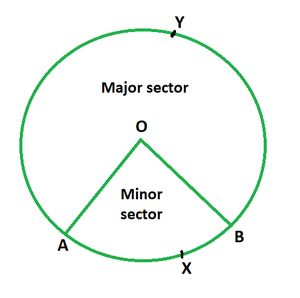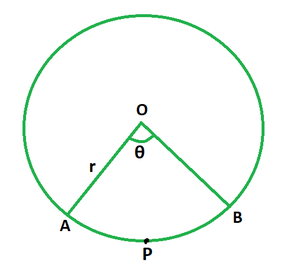GeeksforGeeks App
Open AppBrowser
Continue

# Area of a Sector of a Circle Formula

A sector is a section of a circle bounded by its two radii and the arc that connects them. A semi-circle, which represents half of a circle, is the most frequent sector of a circle. A sector of a circle is a pie-shaped section of a circle formed by the arc and its two radii. A sector is produced when a section of the circle’s circumference (also known as an arc) and two radii meet at both extremities of the arc.In the above diagram, the minor sector is the section of the circle OAXB, whereas the large sector is the portion of the circle OAYB.

### Area of a Sector

The area of a sector of a circle is the amount of space occupied inside a sector of a circle’s border. A sector always begins at the circle’s centre. The semi-circle is likewise a sector of a circle; in this case, a circle has two equal-sized sectors.

Formula

A = (θ/360°) × πr2

where,

θ is the sector angle subtended by the arcs at the center (in degrees),

r is the radius of the circle.

If the subtended angle θ is in radians, the area is given by, A = 1/2 × r2 × θ.

DerivationConsider a circle with centre O and radius r, suppose OAPB is its sector and θ (in degrees) be the angle subtended by the arcs at the centre.

We know, area of the whole circular region is given by, πr².

If the subtended angle is of 360°, the area of the sector is equal to that of whole circle, that is, πr².

Apply unitary method to find the area of sector for any angle θ.

If the subtended angle is of 1°, the area of the sector is given by, πr²/360°.

Hence, when the angle is θ, the area of sector, OAPB = (θ/360°) × πr2

This derives the formula for area of a sector of a circle.

### Sample Problems

Problem 1. Find the area of the sector for a given circle of radius 5 cm if the angle of its sector is 30°.

Solution:

We have, r = 5 and θ = 30°.

Use the formula A = (θ/360°) × πr2 to find the area.

A = (30/360) × (22/7) × 52

= 550/840

= 0.65 sq. cm

Problem 2. Find the area of the sector for a given circle of radius 9 cm if the angle of its sector is 45°.

Solution:

We have, r = 9 and θ = 45°.

Use the formula A = (θ/360°) × πr2 to find the area.

A = (45/360) × (22/7) × 92

= 1782/56

= 31.82 sq. cm

Problem 3. Find the area of the sector for a given circle of radius 15 cm if the angle of its sector is π/2 radians.

Solution:

We have, r = 15 and θ = π/2.

Use the formula A = 1/2 × r2 × θ to find the area.

A = 1/2 × 152 × π/2

= 1/2 × 225 × 11/7

= 2475/14

= 176.78 sq. cm

Problem 4. Find the angle subtended at the centre of the circle if the area of its sector is 770 sq. cm and its radius is 7 cm.

Solution:

We have, r = 7 and A = 770.

Use the formula A = (θ/360°) × πr2 to find the value of θ.

=> 770 = (θ/360) × (22/7) × 72

=> 770 = (θ/360) × 154

=> θ/360 = 5

=> θ = 1800°

Problem 5. Find the area of a circle if the area of its sector is 132 sq. cm and the angle subtended at the centre of the circle is 60°.

Solution:

We have, θ = 60° and A = 132.

Use the formula A = (θ/360°) × πr2 to find the value of θ.

=> 132 = (60/360) × (22/7) × r2

=> 132 = (1/6) × (22/7) × r2

=> r2 = 252

=> r = 15.87 cm

Now, Area of circle = πr2

= (22/7) × 15.87 ×15.87

= 5540.85/7

= 791.55 sq. cm

My Personal Notes arrow_drop_up
Related Tutorials